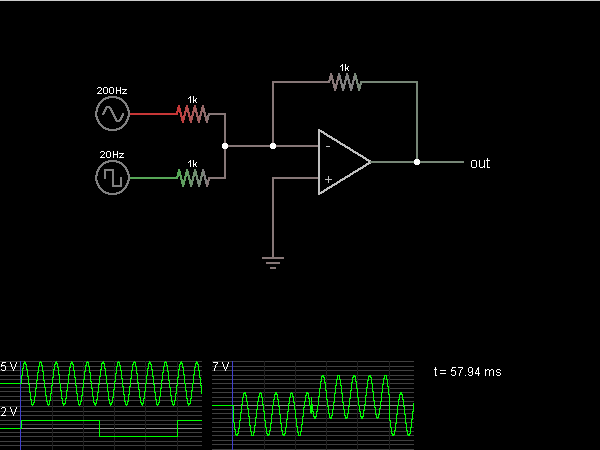# Circuit Simulator - Summing Amplifier

## Where can I get Summing Amplifier Circuit Diagram with Explanation?

IndiaBIX provides you lots of fully solved Summing Amplifier circuit diagram with detailed explanation and working principles.

## How to design a Summing Amplifier (electronic circuit)?

You can easily design the Summing Amplifier circuit by practicing the exercises given below. Here you can design and simulate your own electronic circuits with this Online Circuit Designer and Simulator.### Circuit Description:

This circuit outputs the (inverted) sum of the voltages of two input signals. In this case, the first input is a 200 Hz sine wave, and the second input is a 20 Hz square wave.

The op-amp attempts to keep its – input at the same voltage as the + input (which is at ground). So, both input signals are driving 1k resistors whose other end is at ground. The current across the third resistor is the sum of the two currents, so the voltage drop must be equal to the sum of the two voltage drops, which is the (negative) sum of the input voltages. -- Credits: Mr. Paul Falstad.